﻿ 顶浪规则波中小水线面双体船纵向运动特性数值分析
 舰船科学技术2016, Vol. 38Issue (8): 5-10PDF

Numerical study on characteristics of SWATH ship longitudinal motions in regular head waves
DENG Lei, DONG Wen-cai, YAO Chao-bang
Department of Naval Architecture Engineering, Naval University of Engineering, Wuhan 430033, China
Abstract: Based on RANS equations and VOF method to solve viscous wave flow field and Overset technique to deal with ship motions, this study investigates the characteristics of longitudinal motions for Small Waterplane Area Twin Hulls (SWATH) in regular head waves. The computation method is validated by comprehensive comparison between numerical results and experimental data. Further, the mechanism of peaks in the responses transfer function curves as well as the influence of twin-hull interaction on the motion responses of SWATH are analysed. The results show that the one peak in the responses transfer function curves is caused by the natural resonance of ship, at that moment, the encounter frequency is close to the natural frequency of ship motions. And another peak is probably related to the particular hull form and the appendage configuration of SWATH ship. Influenced by the twin-hull interaction, peaks of motion responses for SWATH have smaller magnitudes and lower frequencies in contrast with that for the single hull.
Key words: SWATH     RANS     motion in waves     characteristics of longitudinal motions     twin-hull interaction
0 引言

1 数值波浪水池 1.1 控制方程及湍流模型

RANS方程是粘性流体运动学和动力学的控制方程，文中以它作为求解船体粘性兴波流场的基本方程。其具体形式如下：

 $\begin{array}{l} \frac{\partial }{{\partial t}}(\rho {u_i}) + \frac{\partial }{{\partial {x_j}}}(\rho {u_i}{u_j}) = - \frac{{\partial p}}{{\partial {x_i}}} + \frac{\partial }{{\partial {x_j}}}\left[{\mu (\frac{{\partial {u_i}}}{{\partial {x_j}}} + \frac{{\partial {u_j}}}{{\partial {x_i}}}) - } \right.\\ \left. {\frac{2}{3}\mu \frac{{\partial {u_l}}}{{\partial {x_l}}}{\delta _{ij}}} \right] + \frac{\partial }{{\partial {x_j}}}( - \rho \overline {{u_i}'{u_j}'} ) + \rho {f_i}, \end{array}$ (1)

1.2 造波与消波

 $\zeta = a\cos (kx - \omega t),$ (2)

 $\left\{ \begin{array}{l} u = a\omega {e^{kz}}\cos (kx - \omega t),\\ v = 0,\\ w = a\omega {e^{kz}}\sin (kx - \omega t) \end{array} \right.$ (3)

2 计算方法验证 2.1 验证对象及流场设置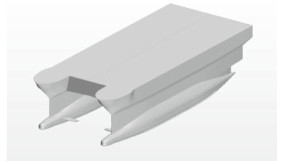图 1 模型三维示意图 Fig. 1 Three-dimensional model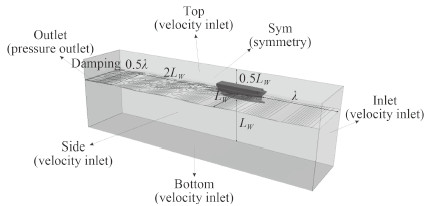图 2 计算流域及边界条件设置 Fig. 2 Computational domain and boundary conditions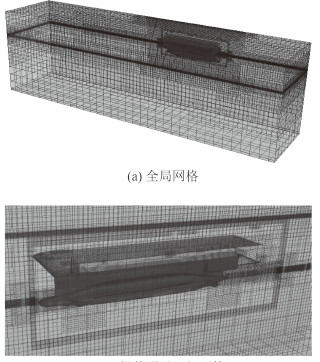图 3 数值模拟网格 Fig. 3 Mesh used for numerical simulations
2.2 计算方法验证表 1 运动响应传递函数定义 Tab.1 The definition of motion transfer functions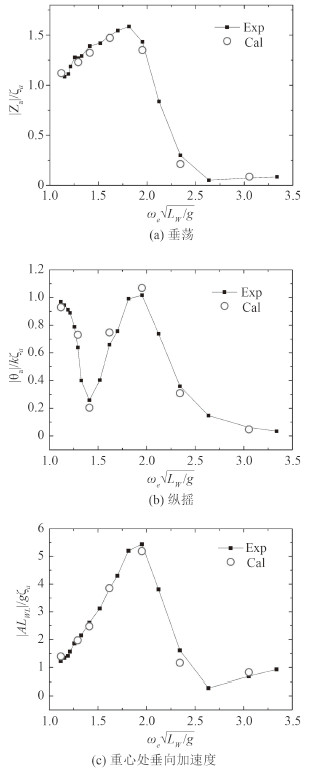图 4 运动响应计算结果与试验结果对比 Fig. 4 Comparison of motion transfer functions between calculated results and experimental data

3 顶浪规则波中SWATH船纵向运动数值分析 3.1 计算对象及工况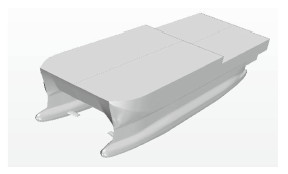图 5 模型三维示意图 Fig. 5 Three-dimensional model
3.2 数值计算结果及分析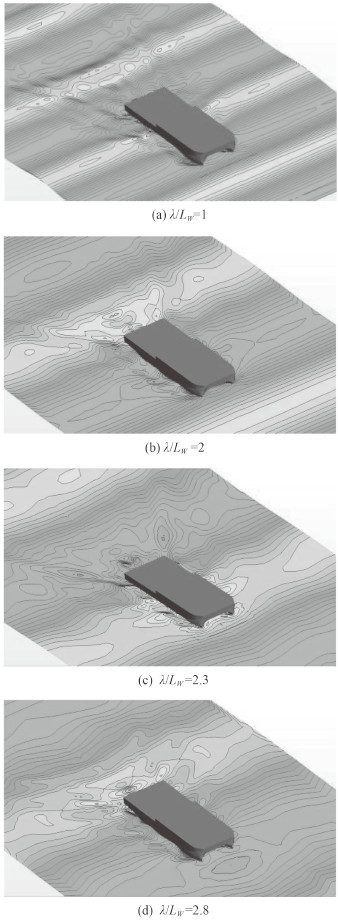图 6 船体在不同波长下自由面波形（Fr=0.21） Fig. 6 Ship motion and free surface at different wave length(Fr=0.21)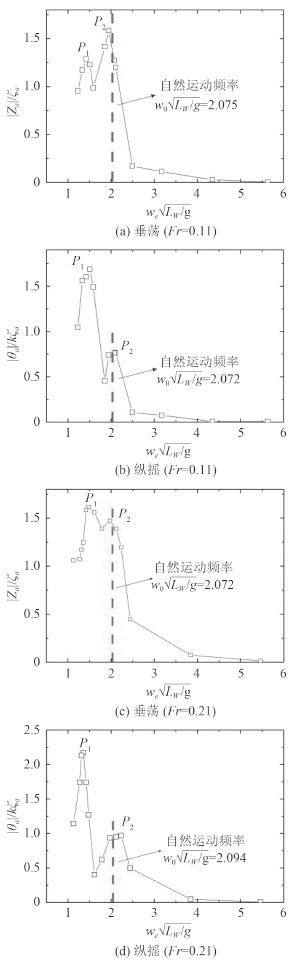图 7 纵向运动传递函数（Fr=0.11，Fr=0.21） Fig. 7 Transfer functions of longitudinal motions(Fr=0.11, Fr=0.21)表 2 船体纵向运动自然频率（Fr=0.11，Fr=0.21） Tab.2 The natural frequencies of ship longitudinal motions(Fr=0.11, Fr=0.21)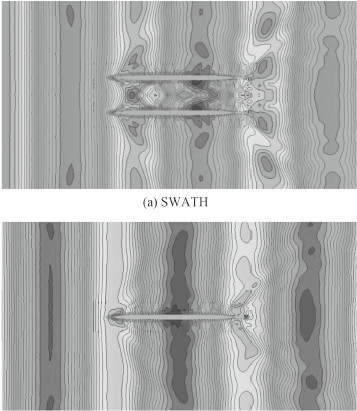图 8 SWATH和SWAMH航行时自由面波形对比（λ/ LW=1） Fig. 8 Comparison of free surface between SWATH and SWAMH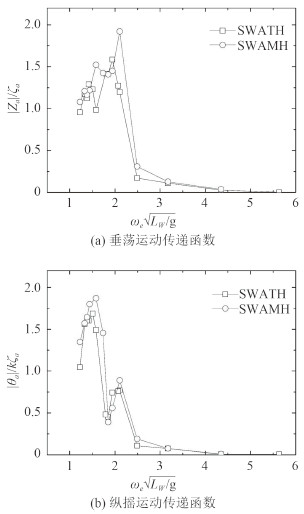图 9 SWATH和SWAMH运动传递函数对比（Fr=0.11） Fig. 9 Comparison of motion transfer functions between SWATH and SWAMH(Fr=0.11)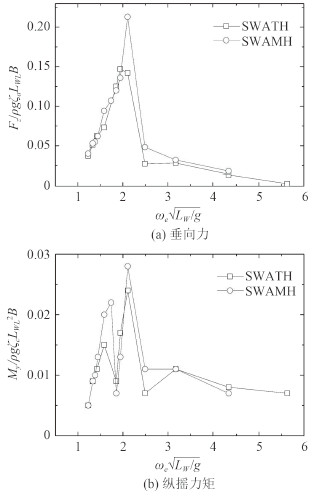图 10 SWATH和SWAMH垂向力和纵摇力矩对比（Fr=0.11） Fig. 10 Comparison of vertical forces and pitchmoments between SWATH and SWAMH(Fr=0.11)

4 结语

1）船体在低频段出现的运动响应峰值P1可能与该SWATH船的船型及附体配置有关；运动响应峰值P2的出现则是因为此时船体遭遇频率接近其运动自然频率，由此发生运动共振。

2）片体间的水动力干扰效应使得SWATH船在波浪中运动响应峰值较单个片体响应峰值明显减小，且出现的位置向低频方向移动。相比于纵摇运动，片体间相互干扰对垂荡运动影响更大。

  黄鼎良. 小水线面双体船性能原理[M]. 北京: 国防工业出版社, 1993 .  董祖舜, 董文才. 小水线面双体船(SWATH)耐波性特点及影响因素分析[J]. 海军工程学院学报 , 1995 (1) :7–15. DONG Zu-shun, DONG Wen-cai. Analysis of some influence factors on seakeeping of small waterplane area twin hull ships (SWATH)[J]. Journal of Naval University of Engineering , 1995 (1) :7–15.  SCHELLINE T E, PAPANIKOLOU A. Prediction of seakeeping performance of SWATH ships and comparison with measurements[C]//FAST' 91, 1st International Conference on Fast sea Transportation. Trondheim, Norway: Tapir Publishers, 811-826.  夏向东.高速小水线面双体船水动力性能研究[D].武汉:海军工程大学, 2011.  BOUSCASSE B, BROGLIA R, STERN F. Experimental investigation of a fast catamaran in head waves[J]. Ocean Engineering , 2013, 72 :318–330.  毛筱菲. 小水线面双体船在波浪中的运动响应预报[J]. 船海工程 , 2005 (4) :13–15. MAO Xiao-fei. Numerical study of the motion response prediction of SWATH ship in waves[J]. Ship & Ocean Engineering , 2005 (4) :13–15.  吴介, 谷家扬, 管义锋, 等. 基于Rankine源法的小水线面双体科考船耐波性预报[J]. 江苏科技大学学报(自然科学版) , 2015, 29 (2) :103–107. WU Jie, GU Jia-yang, GUAN Yi-feng, et al. Prediction of SWATH research ship seakeeping performance based on the Rankine source method[J]. Journal of Jiangsu University of Science and Technology (Natural Science Edition) , 2015, 29 (2) :103–107.  QIAN P, YI H, LI Y H. Numerical and experimental studies on hydrodynamic performance of a small-waterplane-area-twin-hull (SWATH) Vehicle with inclined struts[J]. Ocean Engineering , 2015, 96 :181–191.  SHEN Z R, YE H X, WAN D C. URANS simulations of ship motion responses in long-crest irregular waves[J]. Journal of Hydrodynamics , 2014, 26 (3) :436–446.  LEE C M, CURPHEY R M. Prediction of motion, stability, and wave load of small-waterplane-area, twin-hull ships[C]//SNAME Transactions, 1977, 85: 94-130.  LEE C M. Theoretical prediction of motion of small-wat-erplane-area, twin-hulls (SWATH) ship in waves[R]. David Taylor NSRDC Report, 76-0046, Bathesda (Maryland): DTNSRDC, 1976.  RUSCHE H. Computational fluid dynamics of dispersed two-phase flows at high phase fractions[D]. UK: Imperial College, 2002. http://ethos.bl.uk/OrderDetails.do?uin=uk.bl.ethos.402087  CHOI J, YOON S B. Numerical simulations using momentum source wave-maker applied to RANS equation model[J]. Coastal Engineering , 2009, 56 (10) :1043–1060.  CARRICA P M, WILSON R V, NOACK R W, et al. Ship motions using single-phase level set with dynamic overset grids[J]. Computers & Fluids , 2007, 36 (9) :1415–1433.